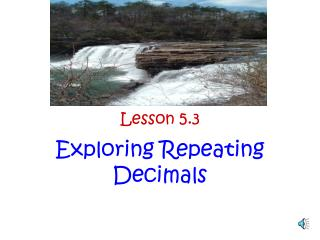Download PresentationLesson 5.3

Lesson 5.3 - PowerPoint PPT Presentation

Lesson 5.3. Exploring Repeating Decimals. ALCOS Standards. PRE-ALGEBRA DAILY PACING GUIDE 2ND QUARTER. Objectives. 1. Students will be able to distinguish between terminating and repeating decimals. 2. Students will be able to identify rational and irrational numbers.I am the owner, or an agent authorized to act on behalf of the owner, of the copyrighted work described.
Download PresentationLesson 5.3

Download Policy: Content on the Website is provided to you AS IS for your information and personal use and may not be sold / licensed / shared on other websites without getting consent from its author.While downloading, if for some reason you are not able to download a presentation, the publisher may have deleted the file from their server.

- - - - - - - - - - - - - - - - - - - - - - - - - - E N D - - - - - - - - - - - - - - - - - - - - - - - - - -
Presentation Transcript
1. Lesson 5.3 Exploring Repeating Decimals

2. ALCOS Standards • PRE-ALGEBRA • DAILY PACING GUIDE • 2ND QUARTER

3. Objectives 1. Students will be able to distinguish between terminating and repeating decimals. 2. Students will be able to identify rational and irrational numbers. • Students will be able to convert decimals to fractions. • Students will be able to identify patterns in repeating decimals.

4. Problem of the Day • What are the decimal representations of 1/33, 2/33, 3/33, and 4/33? • Find other fractions with decimal representations the same as those of 1/33, 2/33, 3/33, and 4/33.

5. Answers to POD • 1/33=.030303… 2/33=.060606… 3/33=.090909… 4/33=.121212… B) 2/66=.030303… 4/66=.060606… 6/66=.090909… 8/66=.121212…

6. Review-Problem 5.2 • Write each fraction as a decimal. Tell whether the decimal is terminating or repeating. If the decimal is repeating, tell which digits repeat. A) 2/3 B) 3/8 C) 5/6 D) 35/10 E) 8/99 *Counting decimal places (power of 10) Place Value: Hands-on role play activity-Counting the Rice http://arcytech.org/java/b10blocks/counting.html

7. Problem 5.2 Answers: • 2/3 =0.666… R • 3/8 =0.375 T • 5/6 =0.8333… R • 35/10 =3.5 T • 8/99 =0.080808… R

8. Repeating Decimals What is a fraction representation of the decimal 0.333…? A) 3/10 B) 1/3 C) 33/100 *Journal: Explain how you got your answer and why the other two are incorrect.

9. Repeating Decimals Correct answer: B)0.333… equals 1/3 Discuss journal entry.

10. Repeating Decimals How could you write the decimal 0.454545… as a fraction?

11. Repeating Decimals In today’s lesson, we will look for patterns that can help us write repeating decimals as fractions. Let’s begin working on the problem (5.3) and follow-up in pairs (pgs. 57-58). Students will break off into pairs.

12. Explore Problem 5.3 Page 57 A, B, C, D *Transparency

13. Explore *A fraction with a denominator of 9 has a decimal representation with a one-digit repeating pattern, or repetend, and the repetend is the number in the numerator. *A fraction with a denominator of 99 has a decimal representation with a two-digit repetend: a 0 followed by the number in the numerator if that number is less than 10; the number in the numerator if that number is equal to or greater than 10.

14. Explore *A fraction with a denominator of 999 has a decimal representation with a three-digit repetend: two 0’s followed by the number in the numerator if that number is less than 10; one 0 followed by the number in the numerator if that number is equal to or greater than 10 but less than 1000; the number in the numerator if that number is equal to or greater than 100.

15. Explore Problem 5.3 Follow Up Page 58 1, 2, 3

16. Follow Up 5.3 Answers: • 1/99=0.0101… 2/99=0.0202… 3/99=0.0303… A fraction with a denominator of 99 is equal to a repeating decimal. The repeating pattern has two digits.

17. Follow Up 5.3 • 1/999=0.001001… 2/999=0.002002… 3/999=0.003003… 10/999=0.010010… A fraction with a denominator of 999 is equal to a repeating decimal. The repeating pattern has 3 digits.

18. Follow Up 5.3 • a) 0.333…=1/3 b) 0.050505…=5/99 c) 0.454545…=45/99 d) 0.045045…=45/999 e) 10.1212…=10 12/99 f) 3.999…=3 9/9, or 4

19. Repeating Decimals • Discuss the patterns in the decimals. *Question: What is the decimal representation for 19/9? For 20/9? *Write 19/9 as a mixed number. *Write 20/9 as a mixed number.

20. Repeating Decimals Question: What fraction is equivalent to 4.111111…? Answer: (write as a fraction and mixed number)

21. Repeating Decimals Answers: 4.111…=37/9 mixed number: 4 1/9

22. Rational / Irrational Numbers Did you know? Refer to text page 58. *http://www.eduplace.com/math/mathsteps/7/a/index.html

23. Rational/Irrational Numbers Math Video Clip: http://www.youtube.com/watch?v=OjjcF0t6CZ0

24. ACE Problems Page 59 # 5 - 8 Pages 61-62 # 26 - 30

25. Homework ACE Problem #25

26. Assessment Teacher Observation Class Discussion / ACE Problems Mathematical Reflections Page 63

27. Additional Resources/Activities *Interactive Fractions to Decimals http://www.learner.org/channel/courses/learningmath/number/session7/part_a/rings.html *A Well Planned Diet http://www.glencoe.com/sec/math/msmath/mac04/course2/webquest/unit3.php/ *Interactive activities: www.aplusmath.com/ *Interactive activities: www.aaamath.com/ *Interactive Baseball Game: http://www.funbrain.com/math/